﻿ SQL Select Statement - TutorialsTrend
This Angular tutorial helps you get started with Angular quickly and effectively through many practical examples.
`

# SQL-Select Statement

In this tutorial introduces you to the basics of the SQL Server Select statement, focusing on how to query SELECT with WHERE, GROUP BY, and ORDER BY against a single table.

#### SQL Server SELECT statement

Database tables are objects that store all the data in a database. In a table, data is logically organized in a row-and-column format. Select is the most commonly used statement in SQL. The SELECT Statement in SQL is used to retrieve or fetch data from a database. We can fetch either the entire table or according to some specified rules.

#### SQL Server Execution Sequence Order

In this case, SQL Server processes the clauses in the following sequence order:

FROM -> WHERE -> GROUP BY -> HAVING -> SELECT -> DISTINCT -> ORDER BYSQL Query mainly works in three phases to execute following clauses.

1. Row filtering - Phase 1: This phase are done by FROM, WHERE , GROUP BY , HAVING clause.
2. Column filtering - Columns are filtered by SELECT clause.
3. Row filtering - Phase 2:  This phase are done by DISTINCT , ORDER BY clause.

We will explain with an example. Suppose we have a students table as follows:

``````CREATE TABLE [students](
[StudentID] [int] IDENTITY(1,1) NOT NULL,
[StudentName] [varchar](100) NULL,
Marks [int],
Section [varchar](100),
[StudentAge] [int] NULL
) ON [PRIMARY]
GO``````

Now insert some data in students table. It looks like that: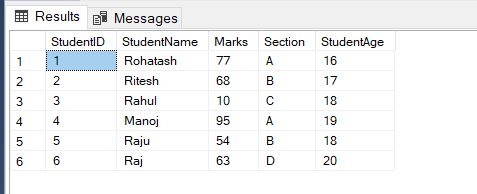#### 1. SQL Server SELECT - Retrieve Some Columns of a Table

The following query finds the StudentName and Marks of all students:

``````SELECT StudentName, Marks
FROM students``````

Here is the result: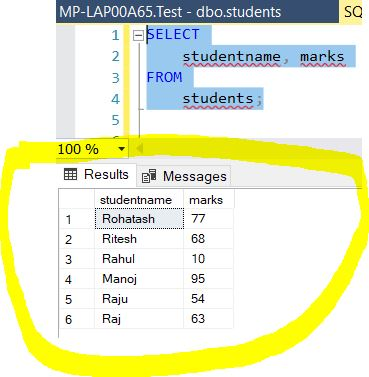#### 2. SQL Server SELECT - Retrieve All Columns of a Table

The following query finds all the columns of students table:

``SELECT * FROM students``

Here is the result: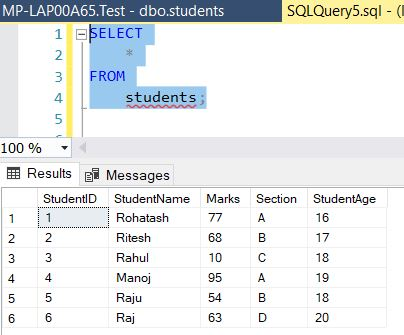Advantage - Retrieve All Columns of a Table

• The SELECT * is helpful in examining the columns and data of a table that you are not familiar with or not used in application.

Disadvantage - Retrieve All Columns of a Table

• SELECT * often retrieves more data than your application needs to function. It causes unnecessary data to transfer from the SQL Server to the client application, taking more time for data to travel across the network and slowing down the application.
• If the table is added one or more new columns, theSELECT * just retrieves all columns that include the newly added columns which were not intended for use in the application. This could make the application crash.

#### 3. SQL Server SELECT - WHERE Clause

To filter rows based on one or more conditions on table, you use a WHERE clause as shown in the following example:

``SELECT * FROM students where Section='A'``

Here is the result: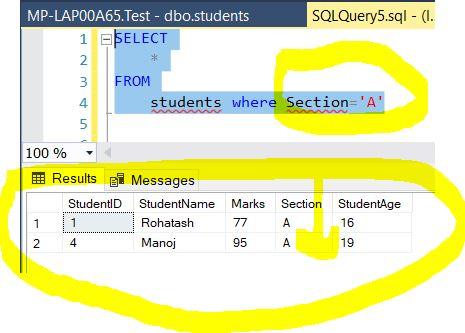#### 4. SQL Server SELECT - ORDER BY Clause

To sort the result set based on one or more columns, you use the ORDER BY clause as shown in the following example:

``````SELECT
*
FROM
students WHERE Section='A' ORDER BY studentName``````

Here is the result: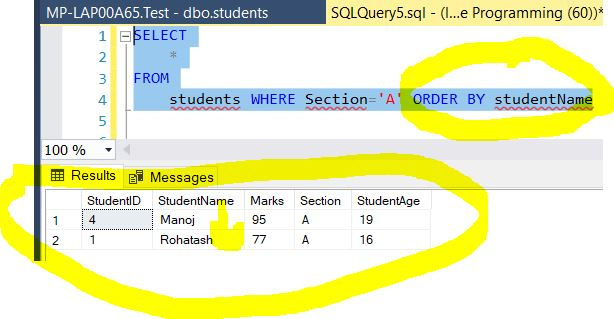#### 5. SQL Server SELECT - GROUP BY Clause

To group rows into groups, you use the GROUP BY clause. you use the GROUP BY clause as shown in the following example:

``````SELECT
studentage,
count(*)
FROM
students where studentage<20 group by student age ORDER BY studentage ``````

Here is the result: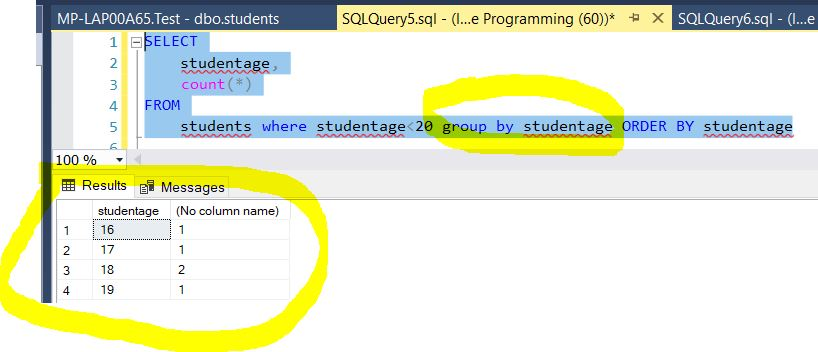#### 6. SQL Server SELECT - Filter Groups

To filter groups based on one or more conditions, you use the HAVING clause. as shown in the following example:

``````SELECT
studentage,
count(*)
FROM
students where studentage<20 group by studentage HAVING COUNT (*) > 1 ORDER BY studentage``````

Here is the result: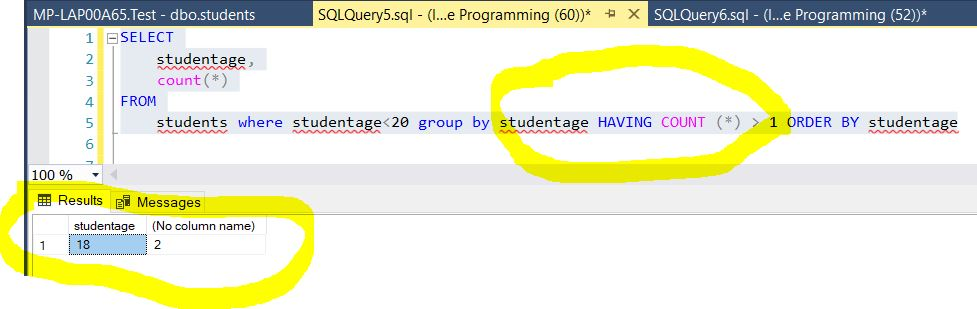Next